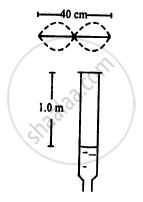Advertisement Remove all ads

# Consider the Situation Shown in Figure.The Wire Which Has a Mass of 4.00 G Oscillates in Its Second Harmonic and Sets the Air Column in the Tube - Physics

Sum

Consider the situation shown in the figure.The wire which has a mass of 4.00 g oscillates in its second harmonic and sets the air column in the tube into vibrations in its fundamental mode. Assuming that the speed of sound in air is 340 m s−1, find the tension in the wire.Advertisement Remove all ads

#### Solution

Given:
Speed of sound in air v = 340 ms−1
Length of the wire l = 40 cm = 0.4 m
Mass of the wire M = 4 g

Mass per unit length of wire $\left( m \right)$ is given by :

$m = \frac{\text { Mass }}{\text { Unit length }} = {10}^{- 2} \text { kg/m }$

$n_0$= frequency of the tuning fork
T = tension of the string
Fundamental frequency : $n_0 = \frac{1}{2L}\sqrt{\frac{T}{m}}$

For second harmonic,

$n_1 = 2 n_0$ :

$n_1 = \frac{2}{2L}\sqrt{\frac{T}{m}} . . . . . \left( i \right)$

$n_1 = 2 n_0 = \frac{340}{4} \times 1 = 85 \text { Hz }$

On substituting the respective values in equation (i), we get :

$85 = \frac{2}{2 \times 0 . 4}\sqrt{\frac{T}{{10}^{- 2}}}$

$\Rightarrow T = (85 )^2 \times (0 . 4 )^2 \times {10}^{- 2}$

$= 11 . 6 \text { Newton }$

Hence, the tension in the wire is 11.6 N.

Concept: Wave Motion
Is there an error in this question or solution?
Advertisement Remove all ads

#### APPEARS IN

HC Verma Class 11, 12 Concepts of Physics 1
Chapter 16 Sound Waves
Q 50 | Page 355
Advertisement Remove all ads
Advertisement Remove all ads
Share
Notifications

View all notifications

Forgot password?